# Running Your First Notebook – Apache SparkThis notebook will show you how to install the course libraries, create your first Spark cluster, and test basic notebook functionality. To move through the notebook just run each of the cells. You will not need to solve any problems to complete this lab. You can run a cell by pressing “shift-enter”, which will compute the current cell and advance to the next cell, or by clicking in a cell and pressing “control-enter”, which will compute the current cell and remain in that cell.

This notebook covers:

• Part 1: Attach class helper library
• Part 2: Test Spark functionality
• Part 3: Test class helper library
• Part 4: Check plotting
• Part 5: Check MathJax formulas

#### (1a) Install class helper library into your Databricks CE workspace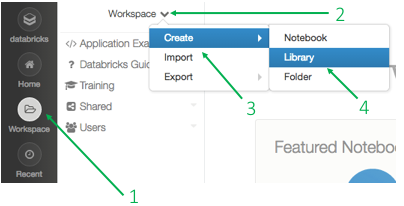• Step 2 Enter the name of the library by selecting “Upload Python Egg or PyPI” and entering “spark_mooc_meta” in the “PyPI Name” field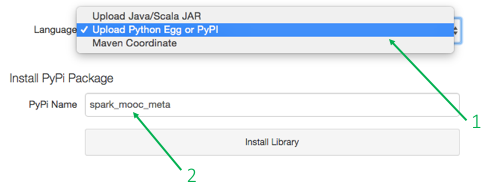• Step 3 Make sure the checkbox for auto-attaching the library to your cluster is selected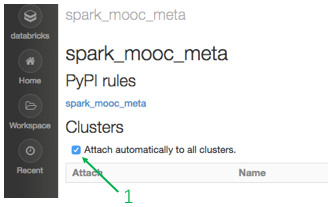#### Part 1: Test Spark functionality

(1a) Create a DataFrame and filter it

When you run the next cell (with control-enter or shift-enter), you will see the following popup.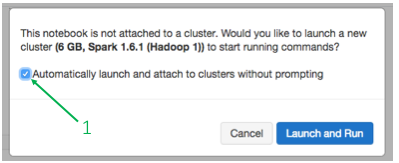Select the click box and then “Launch and Run”. The display at the top of your notebook will change to “Pending”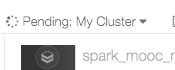Note that it may take a few seconds to a few minutes to start your cluster. Once your cluster is running the display will changed to “Attached”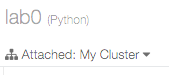Congratulations! You just launched your Spark cluster in the cloud!

# Check that Spark is working
from pyspark.sql import Row
data = [('Alice', 1), ('Bob', 2), ('Bill', 4)]
df = sqlContext.createDataFrame(data, ['name', 'age'])
fil = df.filter(df.age > 3).collect()
print fil

# If the Spark job doesn't work properly this will raise an AssertionError
assert fil == [Row(u'Bill', 4)]
[Row(name=u’Bill’, age=4)]

# Check loading data with sqlContext.read.text
import os.path
baseDir = os.path.join('databricks-datasets', 'cs100')
inputPath = os.path.join('lab1', 'data-001', 'shakespeare.txt')
fileName = os.path.join(baseDir, inputPath)

dataDF = sqlContext.read.text(fileName)
shakespeareCount = dataDF.count()

print shakespeareCount

# If the text file didn't load properly an AssertionError will be raised
assert shakespeareCount == 122395
122395

#### Part 3: Test class testing library

(3a) Compare with hash

Run the following cell. If you see an ImportError, you should verify that you added the spark_mooc_meta library to your cluster and, if necessary, repeat step (1a).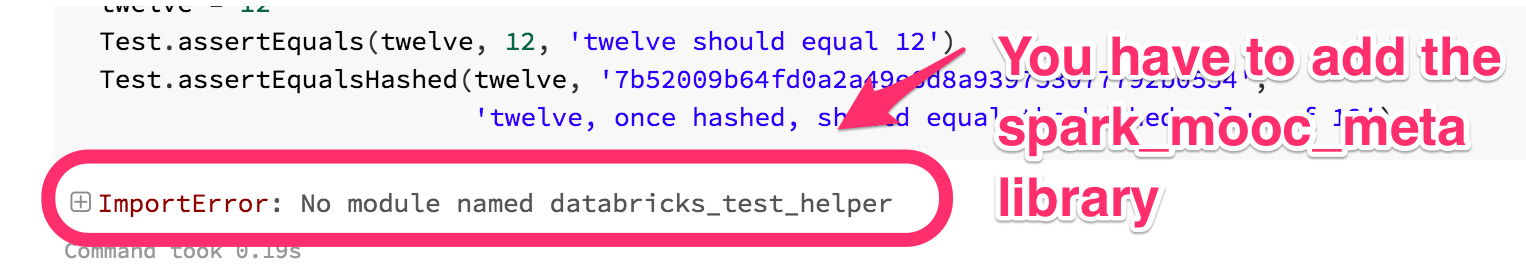# TEST Compare with hash (2a)
# Check our testing library/package
# This should print '1 test passed.' on two lines
from databricks_test_helper import Test

twelve = 12
Test.assertEquals(twelve, 12, 'twelve should equal 12')
Test.assertEqualsHashed(twelve, '7b52009b64fd0a2a49e6d8a939753077792b0554',
                        'twelve, once hashed, should equal the hashed value of 12')
1 test passed. 1 test passed.

(3b) Compare lists

# TEST Compare lists (2b)
# This should print '1 test passed.'
unsortedList = [(5, 'b'), (5, 'a'), (4, 'c'), (3, 'a')]
Test.assertEquals(sorted(unsortedList), [(3, 'a'), (4, 'c'), (5, 'a'), (5, 'b')],
                  'unsortedList does not sort properly')
1 test passed.

#### Part 4: Check plotting

(3a) Our first plot

After executing the code cell below, you should see a plot with 50 blue circles. The circles should start at the bottom left and end at the top right.

# Check matplotlib plotting
import matplotlib.pyplot as plt
import matplotlib.cm as cm
from math import log

# function for generating plot layout
def preparePlot(xticks, yticks, figsize=(10.5, 6), hideLabels=False, gridColor='#999999', gridWidth=1.0):
    plt.close()
    fig, ax = plt.subplots(figsize=figsize, facecolor='white', edgecolor='white')
    ax.axes.tick_params(labelcolor='#999999', labelsize='10')
    for axis, ticks in [(ax.get_xaxis(), xticks), (ax.get_yaxis(), yticks)]:
        axis.set_ticks_position('none')
        axis.set_ticks(ticks)
        axis.label.set_color('#999999')
        if hideLabels: axis.set_ticklabels([])
    plt.grid(color=gridColor, linewidth=gridWidth, linestyle='-')
    map(lambda position: ax.spines[position].set_visible(False), ['bottom', 'top', 'left', 'right'])
    return fig, ax

# generate layout and plot data
x = range(1, 50)
y = [log(x1 ** 2) for x1 in x]
fig, ax = preparePlot(range(5, 60, 10), range(0, 12, 1))
plt.scatter(x, y, s=14**2, c='#d6ebf2', edgecolors='#8cbfd0', alpha=0.75)
ax.set_xlabel(r'$range(1, 50) display(fig) pass), ax.set_ylabel(r’$\log_e(x^2))Tags: